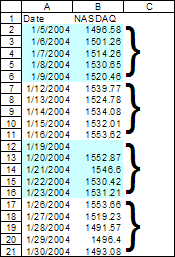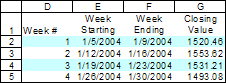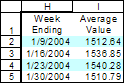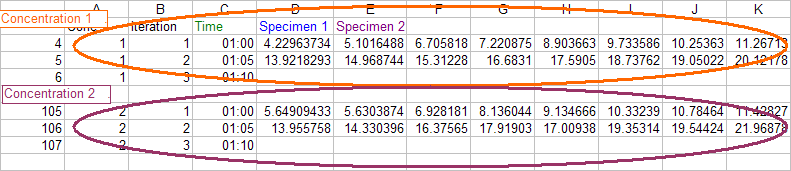You are on the Home/Excel/Excel Tips/Data in Blocks pageShare Your

# Managing data that include logical blocks

There are many instances where a continuous large set of data actually consists of logically blocks.  Such scenarios might include cases such as:

• Each row contains data for a particular day.  Consequently, a block of seven records would correspond to data for one week,
• 12 instruments record data on a periodic basis.  Each row would be the reading of one instrument for one period.  In this case, a set of 12 records would be the block corresponding to one period's readings,
• As shown in Figure 1, the data might represent the closing value of the NASDAQ 100 index each work day.  In this case, each 5 record block represents a work week's worth of data.Figure 1

In these cases, we may need to carry out various operations based on the logical blocks represented in the data.  Examples of such operations would include:

• Calculate the sum (or the average) of the values in each block,
• Get the minimum (or maximum) of the values in each block,
• Find the value of the last entry in each block, or
• Reorganize the data in a cross-tabular form
An example of finding the closing value of the NASDAQ data in Figure 1 might look like:Another example using the same NASDAQ data might be to find the average value for each workweek.  The result would look like:Note that unlike the case on the left, here we don't have a 'week #' column

The theory

Application 1: Calculate the NASDAQ closing quote and average value by week

Application 2: Calculate the total volume by product for each daily 12 hour period for 6 days

Application 3: Isolate titer readings by concentration in a research lab

Using a PivotTable

The theory

Let the number of consecutive records that constitute a logical block be n.  So, in Figure 1 n=5.

Let the record set starts at row r in the worksheet.  Note that this represents the starting row of the first data record and excludes the header.  So, in Figure 1 r=2.

 Convert logical block to data record Convert from data record to logical block Given some block number, x, its starting point within the data set, relative to the start of the data, is given by (x-1)*n. The absolute starting row within the worksheet is r+(x-1)*n. Example: To find the starting point of the data for week 3 (i.e., block # 3) in Figure 1, the calculation would be (3-1)*5=10.  This means that the data for week 3 starts 10 rows from the start of the data set, which in turn, is row 2.  So, the absolute starting row is 2+10 or row 12 of the worksheet. Entry y of block x is located at (x-1)*n+(y-1).  Again, as above, this is relative to the starting point of the data. To calculate the absolute row, use r+(x-1)*n+(y-1). Example: To calculate the worksheet row that corresponds to day 5 of week 3 in Figure 1, one would calculate the starting record of block 3 as indicated above, i.e., (3-1)*5.  Add the zero relative value for day 5 (i.e., 5-1) to get 14.  This means that the data for week 3 day 5 is 14 rows away from the start of the data set.  Since the data set itself starts in row 2, the absolute row number corresponding to Week 3 Day 5 is 2+14=16.  If one were to check Figure 1, row 16 indeed corresponds to Week 3 Day 5. Given a row number z, one can calculate which block it falls in with the formula (z-r) DIV n +1. The record in row 18 of the worksheet in Figure 1 represents an entry in the block given by (18-2) div 5 + 1 or 4.  The corresponding Excel formula would be =DIV(18-2,5)+1 In addition, row z represents a specific entry within the logical block.  This is given by (z-r) MOD n +1. Example:  Row 3 in the worksheet of Figure 1 represents entry number (3-2) MOD 5 +1 = 2 within the logical block that contains row 3.

Application 1: Calculate the NASDAQ closing quote and average value by weekFind the week-by-week closing quote of the index

As shown in Figure 2 (and duplicated in Figure 4), the task we want to show by week number, the starting date, the ending date, and the closing value of the NASDAQ 100 index.  Each week constitutes one logical data block.  The starting date is record 1 of a block, and the ending date and closing value are in record 5 of the block.  As shown in Figure 1, the data table is in columns A and B and starts with row 2

From the section on the theory, recall that record y of block x is in the worksheet row
r+(x-1)*n+(y-1).  In this case, r=2 and n=5.  .

Hence, record one of block x is in row 2+(x-1)*5+(1-1), or 2+(x-1)*5.

Similarly, the last record of block x is in row 2+(x-1)*5+(5-1).

Now, we can create the formula for the starting date of a particular week.  The formula in E2 is
=INDIRECT("A"&(ROW(\$A\$2)+(D2-1)*5),TRUE)
The piece that identifies the row of interest is ROW(\$A\$2)+(D2-1)*5.  To see how this is the same as 2+(x-1)*5, note that

ROW(\$A\$2) gives the starting row of the data table, i.e., r
D2 contains the week number, i.e., x.

Similarly, F2 contains =INDIRECT("A"&(ROW(\$A\$2)+(D2-1)*5)+(5-1),TRUE), where
ROW(\$A\$2)+(D2-1)*5)+(5-1) is the same as 2+(x-1)*5+(5-1)

To get the closing value of the index in F2, all we need to note is that the same method used in F2 applies except that now we want the formula to reference column B rather than column A.  Hence, =INDIRECT("B"&(ROW(\$A\$2)+(D2-1)*5)+(5-1),TRUE)

Calculating the week-by-week average value of the indexAs shown in Figure 3, and duplicated in Figure 5, we want to calculate the average value of the index on a weekly basis.  In addition, this time around, we do not have the very convenient helper column 'Week #'.  That particular column was useful because in gave us the correct block number for our calculations.

However, as convenient as it was, that column was not necessary.  We can calculate the block number with the formula ROW()-ROW(\$H\$2)+1, where H2 is the first row of the summary report.  This is the same as x in the equations above.  Also recall the formula to calculate the row of block x record y relative to the start of the table is (x-1)*n+(y-1).  In this case that becomes (ROW()-ROW(\$H\$2)+1-1)*5+(5-1) or just (ROW()-ROW(\$H\$2))*5+(5-1).

The formula in H2 is =OFFSET(\$A\$2,(ROW()-ROW(\$H\$2))*5+(5-1),0,1,1)

(ROW()-ROW(\$H\$2))*5+(5-1) is the same as (x-1)*5+(5-1).  However, instead of adding the row number of the first row of the data table, we specify it as the first argument to the OFFSET function.

How about the actual formula to calculate the weekly average?  The formula in I2 is =AVERAGE(OFFSET(\$A\$2,(ROW()-ROW(\$H\$2))*5,1,5,1))

The first argument to the OFFSET function is \$A\$2 -- the first cell of the first column of the data table.

The 2nd argument, ROW()-ROW(\$H\$2))*5 calculates the starting row of the block of interest relative to the start of the table.  It is the same as (x-1)*5.

For those interested, the last 3 arguments of the OFFSET function are briefly explained in this paragraph:  These arguments are ...,1,5,1).  The first 1 indicates that we want to refer to the column 1 to the right of the base reference (\$A\$2), i.e., column B.  The 5 and the last 1 indicate that we want to include 5 rows and 1 column in the range created by the function.  So, the complete OFFSET function refers to range 5 rows long by 1 column wide starting with the cell in column B that is (x-1)*5 rows away from the start of the data table (\$A\$2).

Application 2: Calculate the total volume by product for each daily 12 hour period for 6 days

We look at a simplified version of the problem solved at the link above.  The problem involves 25 columns of data that represent 25 different products.  The data, in 72 rows from row 147 to 218, consist of 6 logical blocks each containing 12 rows.  They represent readings over 12 hours for 6 working days each week.  The requirement is to provide a day-by-day summary in a table starting in cell BC3 while leaving a blank row after each set of totals.  So, the 25 cells in row 3 starting with BC3 will have the totals for the first day for each of the 25 products.  The 25 cells in row 5 starting with BC5 will have the totals for the 2nd block of 12 records for each of the 25 products.

In this case, there are two data sets consisting of logical blocks. The raw data has r=147, n=12. The output section has r=3, n=2.

As is often the case in problems of this nature, it is best to start with the output row.  The formula to use in BC3 would be:

=SUM(OFFSET(\$A\$147,(ROW()-ROW(\$BC\$3))/2*12,COLUMN()-COLUMN(\$BC\$3),12,1))

Given a particular output row, we need to find its corresponding data block in the input section. The formula above is (z-r) div n +1
ROW() gives the record number of a particular row in the output section, i.e., z.
ROW()-ROW(\$BC\$3) is the equivalent of (z-r)
ROW()-ROW(\$BC\$3))/2 is the equivalent of (z-r) div n. This is so because the formula is entered only in alternate rows 3, 5, 7, etc.  So,  division by 2 is the equivalent of the DIV function.
ROW()-ROW(\$BC\$3))/2+1 is the equivalent of  (z-r) div n +1.  However, as in Application 1 above, the 1 will be subtracted off soon and won't appear in the final formula.

This gives us the value of x.  Now that we have the block number that the output row corresponds to, we can find the physical record in the input section where this block begins. The formula for the starting row is r + (x-1)*n.  Using the number specific to this problem, we get 147+ ((ROW()-ROW(\$BC\$3))/2+1-1)*12. Note that the +1 and -1 cancel out to give the final result of (ROW()-ROW(\$BC\$3))/2*12. Also, by leaving out the 147 piece, we get a reference that is *zero relative* to the start of the data set. The COLUMN()-COLUMN(\$BC\$3) simply selects the different columns to sum based on the relative distance from column BC.

Now, that we've established the starting row and column *zero relative* to the start of the data set, the OFFSET then uses ,12,1) to select 12 rows and 1 column as the range for the SUM() function.

Application 3: Isolate microtiter readings by concentration in a research lab

This case is adapted from a posting at http://www.mrexcel.com/board2/viewtopic.php?t=95985&start=10

A summary description of the problem:

There is an array of 12 columns (A-L) and 8 rows (starting with 4-11) that represents the wells in the plate. This same array is repeated 100 times with a blank row between each iteration. This represents each reading at increasing time points.  Also on the starting sheet is a row (3) that is 100 columns wide containing the time of the read.
What I need to do now is to take each set of 8 rows (for column A) and transpose them into 8 columns (C-J) so that each row becomes each different time point.  This should be repeated for each of the other columns B-L.

The readings are absorbance readings from a microtiter plate. The plate itself is divided into 12 columns (1-12) by 8 rows (A-H). The samples are arranged so that each row on the plate is a different specimen and each column is a different concentration. In other words row A is all the same specimen but different dilutions whereas row B is a different specimen.
So the original data from the instrument consists of 100 different reads of the same plate. (A4:L902 remember the extra blank row after each set).
In other words the information is three dimensional and we only want to look at two dimensions - rearranging it so that each row is one of the 100 reads and each column is a separate sample. This is why we put each column on a separate sheet so that all the similar dilutions stay together since ultimately we will only choose the optimum dilution to use results from.

The question then is how to transform the data as shown in the Input data below into the layout shown in Output data.While the poster was pursuing a VBA based solution, it should also be apparent that what we have are two lists.

The first list is the input data organized in logical blocks by time.  The starting row for the data was row 4.  Each block of data used 9 rows -- 8 containing data and one empty row.  Basically, r=4 and n=9.

The second list is the output data organized in logical blocks by conentration.  Since it starts in row 4, r=4.  Each concentration needs 100 rows to describe.  Together with the empty row at the end of each group, we get n=101.

Basically, what we need to do is for each output row to identify the matching range segment of the input data and use the TRANSPOSE function on that range.

Suppose the data are in a sheet named Sheet1.

Then, in a 2nd sheet, say, Sheet2...

In row 3 starting with A3 enter the literals: Conc, Iteration, Time, Specimen1, Specimen2, ..., Specimen8.

In A4 enter =IF(MOD(ROW()-4,101)+1=101,"",INT((ROW()-4)/101)+1)

This identifies which concentration each output block contains -- and also the block number of the output data.  The formula puts 1 in column A of the first output block (concentration 1), 2 in the 2nd output block (concentration 2) etc.

In B4 enter =IF(A4="","",MOD(ROW()-4,101)+1)

This simply adds a sequential number from 1 to 100 in each block.  It creates a unique identifier for each record (time snapshot) within each output block.

In C4 enter =IF(A4="","",INDEX(Sheet1!\$3:\$3,Sheet2!B4))

This 'extracts' the correct time value from the data in row 3 of the input worksheet.

In D4:K4 enter the array formula =IF(A4="","",TRANSPOSE(OFFSET(Sheet1!\$A\$4,(Sheet2!B4-1)*9,Sheet2!A4-1,8,1)))

As is often the case, it is easiest to construct the solution starting with the output side.  First, for any given output row, figure out the corresponding input logical block and record number within that block.  Then, map that information into the corresponding row in the input data.  If we look at any output row, the first column identifies the concentration that that row corresponds to.

In the input, each type of concentration is contained in a column -- A, B, C, etc.  Also, in any output row, the 2nd column contains a number from 1 to 100 for the time sequence when the reading was taken.  On the input side, this is exactly how each logical block is laid out.  So, column B gives us the value of what was called x in the section on the theory.  Finally, remember that n=9 -- 8 rows of readings at each time and one empty row).  Hence  (Sheet2!B4-1)*9 is the equivalent of (x-1)*n.

Sheet2!A4-1 identifies which column contains the concentration that corresponds to the output row, and

8 is the number of rows of with data for any one time reading.

The final result is that data for 'concentration 1' are in rows 4:103, for 'concentration 2' in rows 105:204, all the way to concentration 12 in 1115:1214.

Using a PivotTable

As useful as the technique of mapping logical and physical records is, in some instances it might be easier to use a PivotTable to do the analysis.  While PivotTables do have their limitations, some might find one easier to create than to work through the math involved in this analysis.  It will be easiest to use a PivotTable if one were to add a 'key' column to the existing data and use it for the 'row field.'

In the case of the NASDAQ analysis, it would help to add a 'week number' column.

In the case of the micro-titer analysis, it would help to add two columns to the data.  The first would be the 'Reading Time' and the second would be 'Specimen ID.'  Assuming that the concentration columns were labeled C1, C2, etc., and that the blank rows were removed, one could create one cross-tab PivotTable for each concentration with the Reading Time as the row field and the Specimen ID as the column field.

Note that the data layout in the micro-titer case is still not ideally suited for contemporary data analysis tools.  Ideally speaking, the data should be organized in a table with columns labeled Reading Time, Specimen ID, Concentration Level, and Reading.  This would have allowed us to create a single PivotTable that would contain information about all concentration levels and not one per concentration.  But, the reality is that when Excel interfaces with existing equipment -- in a laboratory or a service center or a manufacturing plant -- we have to accept the data as they are organized and not as we might ideally want them to be.  This case study demonstrates how one can adapt Excel to easily and elegantly analyze such data.

Introduction to DIV and MOD and technical note: For those unfamiliar with the DIV and MOD operators, the first, used as a div n , returns the integer part resulting from dividing the positive integer a by the positive integer n (example: 10 div 3 = 3).  The second, used as a mod n, returns the remainder from the same division (example: 10 mod 3 = 1).  In some implementations, such as VBA, the div operator is represented by the reverse slash symbol \ and used as a \ n.  In other instances -- such as with Excel -- these are implemented as functions and written as DIV(a,n) or MOD(a,n).  Finally, while the both DIV and MOD are unambiguously defined when a and n are positive integers, different implementations may confer different interpretations when either a or n or both are negative integers or real numbers.

An array formula is completed not with the ENTER key but the CTRL+SHIFT+ENTER combination.  If done correctly, Excel will display the formula within braces as in Figure 8.  Remember, you should not enter the curly braces yourself!